American Journal of Civil Engineering
Volume 3, Issue 5, September 2015, Pages: 189-193

Study on Roller Compacted Concrete’s Nominal Fracture Toughness Based on Crack-Depth Ratio

Ren Li-li1, Guo Rui-jin2

1Department of Railway Engineering, Liaoning Railway Vacational and Technical College, Jinzhou, China

2College of Civil Engineering, Liaoning University of Technology, Jinzhou, China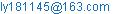(Ren Li-li)

Ren Li-li, Guo Rui-jin. Study on Roller Compacted Concrete’s Nominal Fracture Toughness Based on Crack-Depth Ratio. American Journal of Civil Engineering. Vol. 3, No. 5, 2015, pp. 189-193. doi: 10.11648/j.ajce.20150305.19

Abstract: The roller compacted concrete (RCC) has been widely used, and the practical concrete crack usually has different crack-depth ratios, but the researches about the fracture toughness formula for RCC rarely consider crack-depth ratio. Here the wedge-splitting fracture test was carried on the RCC specimens with different crack-depth ratios(0.4, 0.5, 0.6). At the same time, the theoretical nominal fracture toughness formula based on the crack-depth ratio was also studied. At the end, the modified and suitable RCC' formula based on crack-depth ratio was gotten by combining the RCC test results with theoretical formula.

Keywords: Roller Compacted Concrete, Fracture Toughness, Crack-Depth Ratio, Experiment

1. Introduction

Roller Compacted Concrete (RCC) dam construction technology has been widely used with its advantages such as saving cement, fast construction speed and low engineering cost . But it is worth noting that RCC dam still exists temperature cracks what exist in the normal concrete dam. It is necessary to study the fracture properties of RCC. We have carried on many researches about the RCC and made some achievements in China in the 90 's of the twentieth century[1, 2]. Although there are many aspects researches about the fracture toughness of RCC, and there are also many research about the common concrete fracture toughness considered the crack-depth ratio till now[3-6],but the research about fracture toughness of RCC with different crack-depth ratio is rarely. Here the wedge-splitting fracture test for RCC specimens with different crack-depth ratios is carried out, and analysis the results of the wedge-splitting fracture test. We obtain the fracture toughness formula of RCC considering crack-depth ratio, by combining the experimental results with theoretical formula.

2. The Study on Experimental Fracture Toughness of RCC Specimens

2.1. Experiment Design

2.1.1. Material Selection and Mix Ratio

The experiment use common water from life for test water, P.O 32.5 cement, II grade fly ash, and nature medium sand which requires to particle greater size than 5 mm. Coarse aggregate use the maximum particle size of 20mm. Admixture uses high performance water reducer and the mix ratio of the concrete is shown in the Table 1.

Table 1. Mix ratio of RCC.

 Water Cement Fly ash Sand Gravel Admixture /(Kg/m3) /(kg/m3) /(kg/m3) /(kg/m3) /(kg/m3) /% 130 134 90 805 1280 0.75

2.1.2. Parameters of Specimen and Test Device

The wedge splitting specimen is used. The RCC specimen’s parameters and quantities are shown in table 2. The wedge splitting test is carried out with the 200 kN electro-hydraulic servo testing machine and the constant displacement loading method is used during the test.

Table 2. The parameters of the wedge splitting specimen.

 Specimen type quantity Dimension /mm crack-depth ratio RCC150 14 150×150×150 0.4 6 150×150×150 0.5 6 150×150×150 0.6

2.2. Test Results and Analysis

2.2.1. Selection of Calculation Formula of Fracture Toughness

We use the method in  to analysis. The coordinate system shown in figure 1 (a) is established with the center of the specimen as origin. When left and right symmetrical on both sides of the force system were moved to the B1, B 2 points, the stress system (solid line) of figure1(a) is equivalent as the force system of in figure1(b) of (solid line shows ), where M 4 =1/2Ph D-1/2Pv e according to the force balance shows F=1/2mg+1/2Pv. Using the superposition principle of force, figure1 (b) can be decomposed into figure1 (c) and figure1 (d) cases. According to Saint Venant principle, figure1 (c) can be further equivalent to figure1 (e).The stress situation of the wedge splitting geometry test is transformed for solutions of problems of uniform tensile force P x and pure bending with unilateral openings.

Unilateral opening specimens are subject to axial pull action as shown in figure1 (e) and the stress intensity factor can be calculated by the use of the following formula: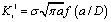(1)

in which,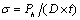,

f(a/D)=1.122-0.231(a/D)+10.550(a/D)2-21.710(a/D)3+30.382(a/D)4.

Unilateral opening specimens are subject to pure bending moment action as shown in Figure 3 (e) and the stress intensity factor can be calculated by the use of the following formula: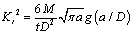(2)

in which,

M=1/2PhD-1/2Pve,g(a/D)=1.122-1.40(a/D)+7.33(a/D)2-13.08(a/D)3+14.0(a/D)4.

Superposition type (1) and (2), when wedge splitting pull geometry is loading at any moment, the expressions of stress intensity factor of crack tip can be expressed as follows: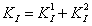(3)

2.2.2. Test Results and Analysis

We can get each test value of nominal fracture toughness , and take the average value are shown in table 3 by using the maximum load of each specimen and original parameter to substitute type (3).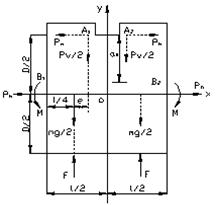(a)                           (b)Fig. 1. Diagram of force on the specimen.

Table 3. Test value.

 Specimen type crack-depth ratio average test value / MPa.m1/2 RCC150 0.4 0.87 0.5 0.76 0.6 0.89

It can be seen that the average value of a high degree of 150 mm of the nominal fracture toughness of the specimen is 0.75~0.9 MPa.m1/2 .m from Figure 4.We can observe the change of fracture toughness with the change of crack-depth ratio, and the fracture toughness of RCC is changed with the change of the crack-depth ratio, but the change rule is not monotonous.

3. Selection of the Formula for the Fracture Toughness of RCC

RCC as a kind of concrete is different from ordinary concrete in the construction process. But studies show that RCC’s size effect rules of fracture toughness are same with ordinary concrete’s. In addition, the RCC is an anisotropic material, the direction of the roller compacted layer is perpendicular to the direction of the crack. The specimens’ force of wedge splitting tensile test is completely symmetrical completely on both sides of the cracks. RCC can be regarded as homogeneous materials along the height direction of each layer, and has same properties with ordinary concrete in this direction. So the RCC maybe have the same nature as the ordinary concrete in the isotropic condition, at the same time due to the different construction process they also have a certain difference. Now the theoretical research on the concrete’s formula of size effect has a lot of, such as Xu Shilang , Bazant, Carpinteri , Hu and Wang.

3.1. Size Effect Formula

3.1.1. Hu’s Size Effect Formula for Fracture Toughness of Concrete 

On the basis of nominal tensile strength size effect formula of concrete, Hu obtained the size effect formula for fracture toughness of concrete: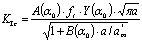(4)

in which: a is crack length; α0 is crack-depth ratio, α0 =a/h;

KIc is nominal fracture toughness; ft is tensile strength;

For three point bending specimen: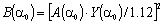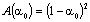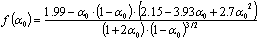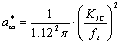in which: KIc is no size effect fracture toughness;

The size effect formula of fracture toughness of the three point bending beam is obtained.(5)

in which: W is specimen height; A1andB1 are the regression parameters are obtained by the test values for a given parameter,

3.1.2. The Modified Formula Based on Hu’s Nominal Size Effect Formula 

Formula (4) is a function of two variables, which are crack-depth ratio and crack length. At the same time, it is also related to the fracture toughness and tensile strength of concrete with no size effect. When the height of the concrete specimen is large enough, the test of fracture toughness value is no longer the size effect. Based on the fracture toughness of concrete without size effect, Wang study the size effect of nominal fracture toughness and get the modified formula: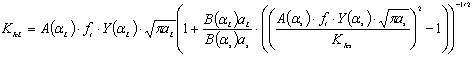(6)

in which: each symbols has the same meaning of various symbols in front.

Compared with formula (5), formula(6) is also considered the effect of crack-depth ratio and crack length. At the same time, it allows the size of the specimens with different crack-depth ratio, has less constraint conditions, less constraint conditions, and has a wide applicable scope. The nominal fracture toughness of the small specimens can be measured easily by the test, and the parameters of the model are not required.

3.1.3. The Nominal Fracture Toughness Size Effect Formula Based on Hu’s Formula

When the formula (5) is simplified further, we can obtain the size effect formula of fracture toughness of concrete containing two coefficients: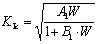(7)

in which: A1andB1 are the coefficient, which are related with α1 B(α1)KIc and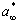It can be determined using the test data of two points.

For formula (7), the fracture toughness is obtained by the two known data points and height of the specimen of the third point, and it is different from conventional size effect formula of the fracture toughness which is obtain from the fracture toughness of known specimens to infer the fracture toughness of unknown specimens, and the test of the small size will get the corresponding fracture toughness usually. In order to obtain the formula of common form, this will change the formula (8)(9).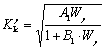(8)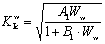(9)

in which: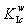is nominal fracture toughness of unknown specimen;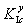is nominal fracture toughness of known specimen, fracture toughness of standard specimen; B1 is related coefficient withα1 ,Bα1and, it can be determined by the test data of two points;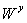is the height of a known specimen;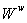is the height of an unknown specimen;

Further, we can obtain the fracture toughness formula which contain the crack-depth ratio and the height.(10)

When the height of the known and the unknown specimen is samewe can obtain Formula (11) only considering the crack-depth ratio.(11)

3.2. Experimental Verification on Fracture Toughness of RCC Considering the Crack-Depth Ratio

We would removal the large deviation value of the test results obtained earlier and use remaining into formula (11). We obtain the theoretical value of the fracture toughness of RCC. As shown in Table 4.

Table 4. Theoretical value of RCC fracture toughness.

 Specimen type crack-depth ratio average test value / MPa.m1/2 theoretical value / MPa.m1/2 RCC150 0.4 0.87 0.87 0.5 0.78 0.85 0.6 0.89 0.84

We can see from table 4 that the theoretical values are in good agreement with the experimental values. It can explain that previous theoretical formula has a certain applicability, but its further law also need to be further explored.

4. Conclusions

We get the following conclusion:

(1) The nominal fracture toughness of the specimen with 150mm height is between 0.75Mpa.m and 0.9Mpa.m1/2.

(2) Under the influence of the crack depth factor, the fracture toughness of RCC is changed, but its change is not monotonous.

(3) We can obtain the fracture toughness formula of RCC , which is usually used to consider the crack depth ratio, by the Hu’s size effect formula:(4) We can get the theoretical values are in good agreement with the experimental values. It can explain that previous theoretical formula has a certain applicability, but its further law also need to be further explored.

Acknowledgements

This work was financially supported by Project of Education Department of Liaoning Province (L2013535).

References

1. Yang Huaquan, Li Wenwei. Research and application of hydraulic concrete[M]. Beijing: China Water Conservancy and Hydropower Press,2005.
2. Zhang Lin, Xu Jin, Chen Xin, et al.Experimental study on fracture of RCC[J]. Journal of water conservancy. 2001, (5):45-49.
3. HU Shao-we,XIE Jian-feng, YU Jiang.Frac ture Toughness ofspecimen withdifferentinitialseam-heightratio underwedgesplittingtest[J]. Journal of Yangtze River Scientific Research Institute, 2015,32(2):114-118.
4. RONG Hua,DONG Wei,WU Zhi-min,FAN Xing-lang.Experimentalinvestigationon double K fracture parameters forlarge initialcrack-depth ratio in concrete[J]. Engineering Mechanics,2012,29(1):162-167.
5. HU Shaowei, MI Zhengxiang.Experimental study on double-K fracture characteristics ofstandard reinforced concrete three-point beam[J]. Journal of Building Structures,2013,34(3):152-157.
6. HU Xiao-wei1,ZHANG Xiu-fang1,XU Shi-lang.Determination of double-Kfracture parameters of concrete using split-tension cubes[J]. Journal of Hydraulic Engineering,2012(S1):98-109.
7. Xu Shilang, Zhang Xiufang, Gao Hongbo. Concrete wedge splitting specimens of double K fracture parameters superposition calculation and boundary effect[J].Journal of Dalian University of Technology, 2006,46(6):867-874.
8. TADA H,PARIS P IRWIN G. The stress analysis of cracks handbook, (2nd,edn)[M].St.Louis:Paris Productions Incorporated,1985.
9. BAZANT Z P. Size effect in blunt fracture:concrete,rock,metal[J].Journal of Engineering Mechanics,1984,110(4):518-535.
10. CARPINTERI A,CHIAIA B,FERRO G. Size effects on nominal tensile strength of concrete structures:multifractality of material ligaments and dimensional transition from order to disorder[J].Materials and Structures,1995,28(180):311-317.
11. DUAN K,HU X Z,WITTMANN F H. Size effect on fracture resistance and fracture energy of concrete[J].Materials and Structures,2003,36:74-80.
12. Wang Xuezhi, BiZhong, Zhang Xiaogang, et al. Experimental study on the size effect of the fracture toughness of RCC[J].Journal of Wuhan University of Technology,2007,29(6):54-57.

 Contents 1. 2. 2.1. 2.2. 3. 3.1. 3.2. 4.
Article ToolsAbstractPDF(445K)